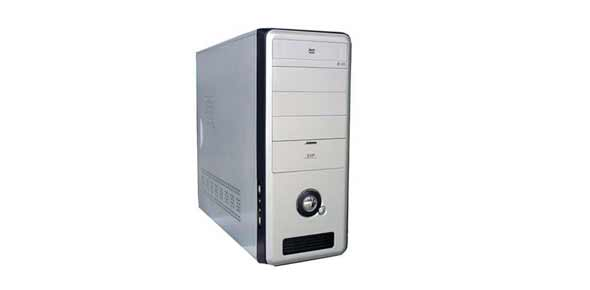# Cccklc003 – CPU

17 Questions | Total Attempts: 224SettingsCCCKLC003 - CPU

Related Topics
• 1.
Which component is called the ‘brain’ of a computer?
• A.

Peripheral

• B.

Main memory (RAM)

• C.

CPU

• D.

ALU

• 2.
Which of the followings is NOT the function of the central processing unit?
• A.

Executes computer instructions

• B.

Processes data

• C.

Stores programs and data permanently

• D.

Communicates with other devices to perform tasks

• 3.
What is an ALU?
• A.

Aero Linguistic Utility

• B.

Arithmetic and Logic Unit

• C.

Another Liberal University

• 4.
Which part of the CPU is responsible for the decoding of computer instructions?
• A.

Arithmetic and logic unit

• B.

Control Unit

• C.

Registers

• D.

Bus line

• 5.
In a CPU machine cycle, ‘fetch’ is the step which ____________
• A.

Get the program/data from main memory

• B.

Translate the program to code which the computer could understand

• C.

Execute the command

• D.

Write the execution result back to RAM

• 6.
In a CPU machine cycle, ‘decode’ is the step which ____________
• A.

Get the program/data from main memory

• B.

Translate the program to code which the computer could understand

• C.

Execute the command

• D.

Write the execution result back to RAM

• 7.
In a ‘machine cycle’ the CPU ‘run’ instructions.  What is the sequence of steps?
• A.

Fetch -> Execute -> Decode -> Store

• B.

Decode -> Execute -> Fetch -> Store

• C.

Fetch -> Decode -> Execute -> Store

• D.

• 8.
In a CPU machine cycle, which of the following step involves the ALU?
• A.

Fetch

• B.

Decode

• C.

Execute

• D.

Store

• 9.
In a CPU machine cycle, which of the following step does NOT involve the CU (Control Unit)?
• A.

Fetch

• B.

Decode

• C.

Execute

• D.

Store

• 10.
In a CPU machine cycle, the CPU retrieve/get computer instruction/program from ___________ .  After execution, the CPU store the result to ____________ .
• A.

Control Unit; Control Unit

• B.

Main memory; main memory

• C.

ALU; CU

• D.

RAM; ROM

• 11.
In a computer, we can describe the ‘bus’ as:
• A.

A vehicle for transportation

• B.

A common device which connect the computer to external networks

• C.

Physical lines which connects CPU and other devices

• D.

A type of twisted-pair cable

• 12.
CPU controls all other components in the computer.  True or false?
• A.

True

• B.

False

• 13.
The memory unit(s) inside a CPU is/are called ____________ .
• A.

RAM

• B.

Main memory

• C.

Registers

• D.

ALU

• 14.
Which of the followings is not a type of bus?
• A.

• B.

Memory bus

• C.

Control bus

• D.

Data bus

• 15.
A computer system can have more than one CPU.  True or false?
• A.

True

• B.

False

• 16.
Use the internet to search for the following information (e.g. go to www.wikipedia.org ): “Intel Pentium D” is a type of CPU.  Its maximum clock rate is ___________ and word size is ____________ .
• A.

3.73 GHz; 32 bits

• B.

3.8 GHz; 32 bits

• C.

3.73 GHz; 64 bits

• D.

3.8 GHz; 64 bits

• 17.
Use the internet to search for the following information (e.g. go to www.wikipedia.org ): Which of the followings is NOT a CPU?
• A.

Intel Atom N470

• B.

GeForce 6200

• C.

AMD Phenom II